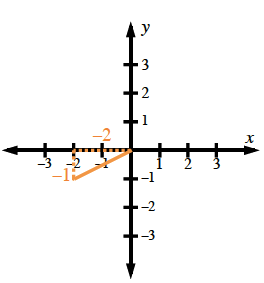### Home > INT3 > Chapter 10 > Lesson 10.1.5 > Problem10-78

10-78.

If $\tan(θ)=\frac{1}{2}$ and $\pi\leθ\le\frac{3\pi}{2}$, what is the value of $\text{sin}\left(θ\right)$?

Draw a diagram.